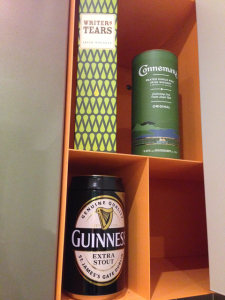# Codility & Intercom

Last months have been trying to seek a career change. Among different job interviews and screenings I got to know also Intercom. It left an awesome and bitter experience. I did manage to go to the on-site interview but I did not get an offer (sigh)… but that story would belong to another post.I spent three days in Dublin and had a chance to make a second visit to the city. All is left from this trip are the souvenirs on the left, which I’ll get rid very quickly 🍻 (I could not find Programmer’s Tears whiskey), and the preparation with Codility Lessons. These lessons consist in various algorithm solving problems which in my case helped me a lot to polish some CS algorithm concepts. I solved all ‘Painless’ and most of ‘Respectable’ ones by myself, and noticed that in particular the solution of two problems could not be found online. So I just wanted to publish my solution to the problems of Sieve of Eratosthenes lesson. Unfortunately I cannot post the problems because they are subject of copyright by Codility. Both solutions get 100% score on correctness and performance. Please find the spartan explanation as comments between source code lines.

### CountSemiprimes

``````class Solution {
public int[] solution(int N, int[] P, int[] Q) {
//the first semi prime is number 4
if (N <= 3){
return new int;
}
// build the sieve of primes up to N/2. Since
// the smallest prime is 2, we cannot consider
// primes bigger than N/2 because the product
// with 2 would result bigger than N.
boolean[] primes = new boolean[N/2 + 1];
for (int i = 0;i <= N / 2;i++){primes[i] = true;}
primes = false;
primes = false;
int i = 2;

while (i * i <= N / 2){
if (!primes[i]){
i++;
continue;
}
int k = i * i;
while(k <= N / 2){
primes[k] = false;
k += i;
}
i++;
}

// build another sieve for semi primes now
boolean[] semiPrimes = new boolean[N + 1];

for (int j = 1; j <= N/2; j++){
if (primes[j]){
int k = j;
// be aware here, the product may overflow
// resulting in a negative number that passes
// the check to be smaller than N
long product = (long)k * j;
while(product <= N){
if (primes[k]){
semiPrimes[j*k] = true;
}
k++;
product = (long)k * j;
}
}
}
//use prefix sum to count the semi primes
//in the required queries.
int[] prefix_sum = new int[N + 1];

int sum = 0;
for (int j = 0; j <= N;j++){
if (semiPrimes[j]){ sum++; }
prefix_sum[j] = sum;
}
int[] result = new int[P.length];
for (int j = 0; j < P.length; j++){
result[j] = prefix_sum[Q[j]] - prefix_sum[P[j]-1];
}
return result;
}
}
``````

### CountNonDivisible

``````class Solution {
public int[] solution(int[] A) {
//each element A is within the range [1..2 * N]
int[] factors = new int[A.length * 2 + 1];

//count how many times each factor appear
for (int i = 0;i < A.length; i++){
factors[A[i]]++;
}

//build a sieve which saves all the common
//multiples smaller than 2 * N of the input factors
int multi[] = new int [factors.length];

for (int i = 1;i < multi.length;i++){
if (factors[i] == 0)
continue;
int k = 1;
long product = (long)i * k;
while(product < multi.length){
//we increase the number of multiples
//by the number of factors in the input
//array
multi[k * i] += factors[i];
k++;
product = (long)i * k;
}
}
//for each input element the number of non divisors
//is the difference of the total number of elements
//and the number of times that element appears as a
//multiple of other input elements
int result[] = new int[A.length];
for (int i = 0;i < A.length; i++){
result[i] = A.length - multi[A[i]];
}
return result;
}
}
``````

Any suggestion is more than welcome! Happy coding!!!

ap